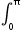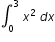# MA.912.C.4.1

Interpret a definite integral as a limit of Riemann sums. Calculate the values of Riemann sums over equal subdivisions using left, right and midpoint evaluation points.

### Examples

Example: Find the values of the Riemann sums over the interval [0,1] using 12 and 24 subintervals of equal width for f(x)=ex evaluated at the midpoint of each subinterval. Write an expression for the Riemann sums using n intervals of equal width. Find the limit of this Riemann Sums as n goes to infinity.

Example: Estimatesin x dx using a Riemann midpoint sum with 4 subintervals.

Example: Find an approximate value forusing 6 rectangles of equal width under the graph of f(x)=x2 between x=0 and x=3. How did you form your rectangles? Compute this approximation three times using at least three different methods to form the rectangles.

General Information
Subject Area: Mathematics (B.E.S.T.)
Strand: Calculus
Status: State Board Approved

## Related Courses

This benchmark is part of these courses.
1202300: Calculus Honors (Specifically in versions: 2014 - 2015, 2015 - 2022, 2022 and beyond (current))

## Related Access Points

Alternate version of this benchmark for students with significant cognitive disabilities.

## Related Resources

Vetted resources educators can use to teach the concepts and skills in this benchmark.

## Student Resources

Vetted resources students can use to learn the concepts and skills in this benchmark.

## Parent Resources

Vetted resources caregivers can use to help students learn the concepts and skills in this benchmark.